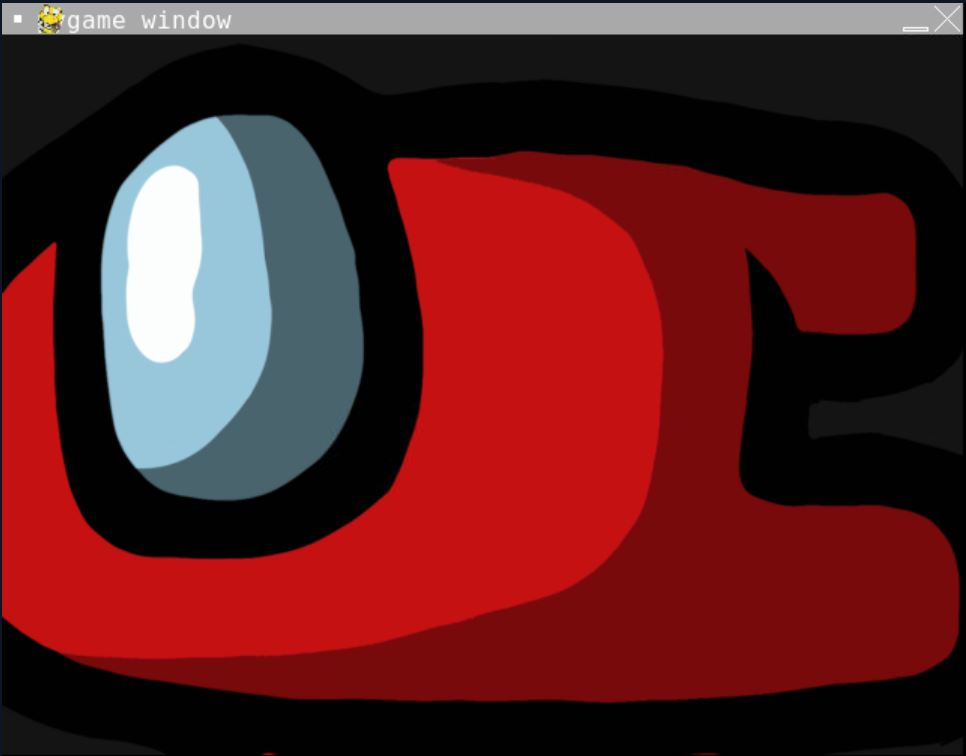# Rotate Images in Pygame

Maxim Maeder May 11, 2022

This tutorial teaches you how to rotate images in Pygame.

## Rotate Images in Pygame

Rotating images and surfaces in Pygame is easy using the `transform.rotate` method. Like the scale method, this will return a new Surface.

We first have to provide the surface, which will be rotated and then the rotation in degrees.

``````# Before the main loop
image = pygame.transform.rotate(image, 45)

# in the main loop
screen.blit(image, (30, 30))
``````

If you rotate a surface in the main loop, it is best to not overwrite the old surface but instead make a new one, like in the code below.

``````rotation += 1
shown_image = pygame.transform.rotate(image, rotation)

screen.blit(shown_image, (30, 30))
``````

## Centered Rotate Image in Pygame

You’ll notice that the rotation is around the top left corner, but you are most likely to want it to rotate around its center. The code below does just that.

``````rotated_image = pygame.transform.rotate(image, 45)
new_rect = rotated_image.get_rect(
center = image.get_rect(
center = (300, 300)
).center
)

screen.blit(rotated_image, new_rect)
``````

Complete Code:

``````# Imports
import sys
import pygame

# Configuration
pygame.init()
fps = 60
fpsClock = pygame.time.Clock()
width, height = 640, 480
screen = pygame.display.set_mode((width, height))

rotation = 0

# Game loop.
while True:
screen.fill((20, 20, 20))
for event in pygame.event.get():
if event.type == pygame.QUIT:
pygame.quit()
sys.exit()

rotated_image = pygame.transform.rotate(
image,
90
)

new_rect = rotated_image.get_rect(
center = image.get_rect(
center = (300, 300)
).center
)

screen.blit(rotated_image, new_rect)
pygame.display.flip()
fpsClock.tick(fps)
``````

Output:Author: Maxim Maeder

Hi, my name is Maxim Maeder, I am a young programming enthusiast looking to have fun coding and teaching you some things about programming.

GitHub